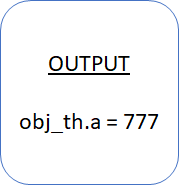## Concept of “THIS” in System Verilog# Concept of “THIS” in System Verilog

Concept and usage of “this” is simple but important in test bench development using system verilog. I would like to share some insights on this concept.

The this keyword is an implicit argument to a method that refers to the current object.

Let’s understand this concept with the following example.

``````class concept_this ;
int a ;
function new (int a);
this.a = a;
endfunction : new
endclass
program main;
concept_this obj_th = new (777);
initial begin
\$display ("obj_th.a = %d", obj_th.a);
end
endprogram
``````In above example we can see that ‘a’ is a property of class “concept_this”. When we initialize the memory for class, we have passed an integer value ‘777’ to its class constructor i.e. function new(). The variable ‘a’ is local to class instance “obj_th and is now 777 as we have passed this from it’s class constructor.

Latest Posts

Hot items

• 0.00